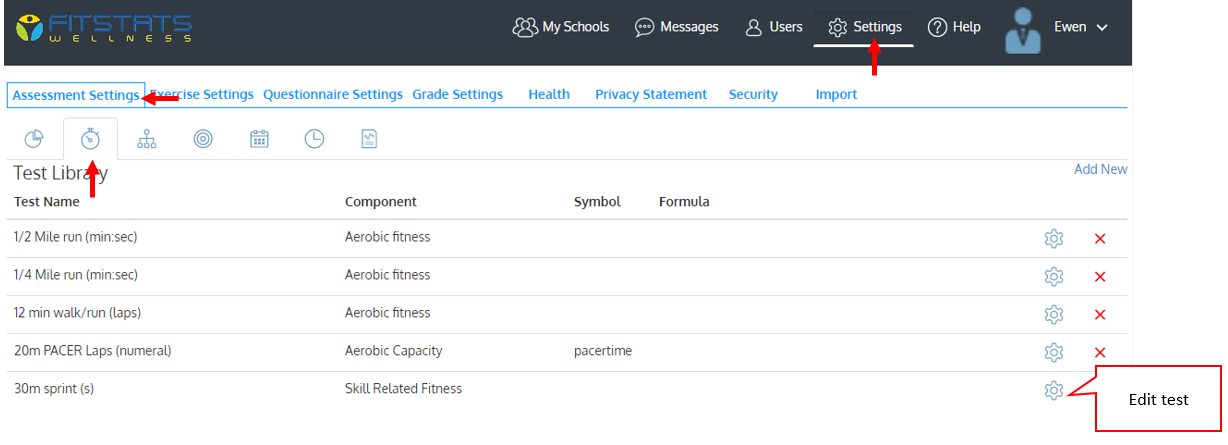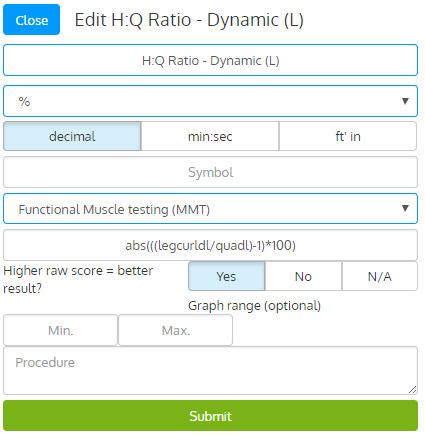### Opening the test library### Using formulas

You can create and use your own formulas to calculate test results. This section presents the operators, functions, constants and variables that you can use in formulas and provides you with examples.### Math operators

- (substraction)
/(division)
* (multiplication)
^ (power)
() parenthesis

### Logical operators

> (greater than)
< (less than)
<= (less than or equal)
>= (greater than or equal)
== (equal)

### Built-in variables

age=student age calculated using the student birth date, according to the information stored in the student list.
gender=student gender according to the information stored in the student list
Any symbol available in the Symbol box of any test of the test library

### Mathematical Functions

FITSTATS  uses a powerful mathematical library that includes many functions that you can use in formulas. For a complete list, click here . All functions listed can be used in FITSTATS formulas.

### Example 1: A simple formula to calculate Body Mass Index

(bweig/bheig^2)*703

bweig=symbol associated with the test named ‘body weight’

bheig =symbol associated with the test named ‘body height”

^=the operator used to calculate bheig to the power of 2

### Example 2: A complex formula that calculates VO2max from one mile walk/run

132.853-(0.1692*(bweig*2.2))-(0.3877*age)+(6.315*gender)-(3.2649*(mitime/60))-(0.1565*ehr)

bweig=symbol associated with the test named ‘body weight’

age =symbol associated with the age of the student (calculated dynamically using the date of birth)

gender= symbol associated with the gender (male/female) of the student

mitime= symbol associated with the test named ‘Mile Rockport Walk Test’; entered in min:sec, automatically converted in seconds when stored in the database and divided by 60 to be used as min in the formula;

ehr=exercise heart rate at the end of the walk/run

Operators -, *, + and / are also used.

Important

Symbols should only contain lowercase letters (eg: a symbol such as "20mSprint" will not work but "twmsprint" will).

### Specifying smallest worthwhile changes

New research recommends using a fraction or multiple of the individual results' standard deviation (SDx0.1 to SDx2) as the smallest meaningful /worthwhile change (SWC).

You can specify the formula that will be used to calculate the test's SWC when creating or editing a test.  This calculation is used to assign white, red and green color on individual assessment graphs, individual profiles and when test results are displayed on the team dashboard.